# Burnside problem

 Importance: Outstanding ✭✭✭✭
 Author(s): Burnside, William
 Subject: Group Theory
 Keywords:
 Recomm. for undergrads: no
 Posted by: dlh12 on: April 11th, 2008
Conjecture   If a group hasgenerators and exponent, is it necessarily finite?

It is possible to define the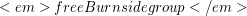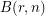to be the group generated bywith relations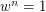whereranges over every word in the generators. There is a universality property: Any homomorphismwherehas r generators and exponent dividingcan be written as a composition of a homomorphismwith a homomorphism. Some cases of this are known:is a cyclic group of order, for any positive integer.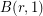is trivial for any positive integer.is isomorphic to the Cartesian product ofcyclic groups of order, for any positive integer. This is because the relations make it easy to prove that the generators commute.is a finite group, and its order isis a finite group, and its order is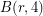is a finite group for any positive integer. The order is known forup to: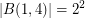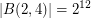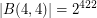is known to be infinite for sufficiently largeand odd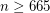, as well asand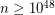divisible by.

Burnside_problemBurnside Problem -- from Wolfram MathWorld

## Bibliography

* indicates original appearance(s) of problem.